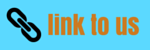# CBSE Important Questions(Long questions) for Work And energy

Notes Assignment NCERT Solutions

Given below are the Class 9 Science Important Questions for work and energy
a) Concepts questions
b) Calculation problems
c) Multiple choice questions
d) Long answer questions
e) Fill in the blank's
1. A light and a heavy object have the same momentum. Find out the ratio of their kinetic energies. Which one has a larger kinetic energy?
2. An object with 100 N weights is raised to a height of 15m. Find the potential energy possessed by the object at that height. Also find the new potential energy:
a) If the same object is raised half of its original height.
b) If the same object is raised to three times of its original height?
3. Define one joule of kinetic energy?
4. A ball of mass 2kg is thrown up with a speed of 10m/s. find the kinetic energy of the ball at the time of throwing. Also find the potential energy of the ball at the highest point?
5. An object of mass m and velocity v has kinetic energy= 200J. Find the new kinetic energy if the mass of the object becomes double and velocity still remains the same?
6. Calculate the kinetic energy of a car of mass 500kg moving with a velocity of 36km/h. Find the kinetic energy if the velocity of car doubles?
7. Calculate the power of an electric motor that can lift 800 kg of water to store in a tank at a height of 1500cm in 20s. (g=10m/s2).
8. Calculate the electrical energy consumed in Joules if a toaster of 60 W is used for 30 minutes?
9. An electric heater of 1000 W is used for two hours in a day? What is the cost of using it for a month of 28 days, if one unit costs 3.00 rupees?
10. What energy transformation takes place in :
a) Dry cell
b) electric fan

11. Calculate the electricity bill amount for a month of 30 days, if the following devices are used as specified:
a) two bulbs of 40W for six hours.
b) two tubelights of 50W for eight hours
c) A TV of 120W for six hours.
d) Give the rate of electricity is 2.50 rupees per unit?
12. A crane pulls up a car weighing 500kg to a vertical height of 4m. Calculate the work done by the crane?
13. A lamp consumes 500 J of electrical energy in 20 sec. What is the power of the lamp?
14. In a factory four bulbs of 100 W each and five fans of 110 W each operate for 12 hours daily. Calculate the units of electricity consumed? Also find the total expenditure if one unit costs 2.50 rupees?
15. The work done in lifting a box on to a plateform does not depend upon how fast it is lifted up. Explain your answer giving proper reason?
16. A geyser of 2.5kW is used for eight hours daily? Calculate the monthly consumption of electrical energy units. Also calculate the cost of electrical units consumption in a month if rate per unit is 3.50 rupees?
17. Two bodies of equal masses move with the uniform velocities v and 3v respectively. Find the ratio of their kinetic energy?